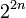# Difference between revisions of "Quiz:Degrees of irreducible representations"

## Find the feasible or infeasible degrees of irreducible representations

1 Which of the following is not a possibility for the multiset of the degrees of irreducible representations of a finite group over a splitting field of characteristic zero (such as the complex numbers)?

 1,1,1,1,2 1,1,1,1,3 1,1,1,1,4 None of the above, i.e., they are all possibilities for the multiset of degrees of irreducible representations.

2 Which of the following is not a possibility for the multiset of the degrees of irreducible representations of a finite group over a splitting field of characteristic zero (such as the complex numbers)?

 1,1,2,3,3 1,1,1,2,2,2,3 1,1,1,1,2,2,2,2,2 1,1,1,1,1,1,1,2,2,3 1,1,1,1,1,1,1,1,2,2,2,2

3 Which of the following is not a possibility for the multiset of the degrees of irreducible representations of a finite group over a splitting field of characteristic zero (such as the complex numbers)?

 1,1 1,1,2 1,1,1,3 1,1,1,1,4 None of the above, i.e., they are all possibilities

## Maximum and divisibility

For all the questions below, we consider irreducible representations over a splitting field of characteristic zero, such as the field of complex numbers.

1 Which of the following statements is false in general about the degrees of irreducible representations of a finite group over a splitting field of characteristic zero?

 The degree of any irreducible representation divides the index of any abelian subgroup in the group. The degree of any irreducible representation is bounded by, but need not divide, the index of any abelian subgroup in the group. The degree of any irreducible representation divides the index of any abelian normal subgroup in the group.

2 What is the largest possible value of the maximum degree of irreducible representation for a group of order 24 over a splitting field of characteristic zero (such as the complex numbers)?

 2 3 4 6 8

3 What is the largest possible value of the maximum degree of irreducible representation for a group of order$2^{2n + 1}$ over a splitting field of characteristic zero (such as the field of complex numbers) where$n$ is a positive integer?

 2$2^n$$2^{n + 1}$$2^{2n - 1}$$2^{2n}$$2^{2n + 1}$

4 It is true in general that degree of irreducible representation divides index of abelian normal subgroup, when we are working with irreducible representations of finite groups over splitting fields of characteristic zero. Which of the following gives an example of a group where the least common multiple of the degrees of irreducible representations is strictly smaller than the greatest common divisor of the index values of all abelian normal subgroups?

## Groups of prime power order: smallest counterexamples

1 What is the smallest power$2^n$ such that there exist two groups of order$2^n$ of the same nilpotency class but with different multisets of degrees of irreducible representations over the field of complex numbers?

 8 16 32 64 128

2 What is the smallest power$2^n$ such that there exist two groups of order$2^n$ with the same multiset of degrees of irreducible representations over the field of complex numbers but such that the conjugacy class size statistics are different for the groups?

 8 16 32 64 128

3 What is the smallest power$2^n$ such that there exist two groups of order$2^n$ with the conjugacy class size statistics but such that the degrees of irreducible representations are different for the groups?

 8 16 32 64 128